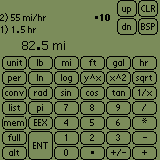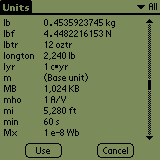# Unc: A Units Calculator

## and for Microsoft WindowsUnc is a calculator which runs on the 3Com PalmPilot and on Microsoft Windows. Unc has a number of features not often found in other calculators:

• Units. One of Unc's main features is its ability to calculate using units, like feet, miles, pounds, meters, seconds, and so on. Unc does not merely convert between units (although it certainly does conversions), but also performs calculations on numbers containing units. For example, you can multiply "55 miles per hour" by "1.5 hours" and get the result "82.5 miles". Unc already knows most of the units in common use as well as specialized units used in various fields. You can also define your own units and Unc will use them in calculations just like the built-in units.

• Arithmetic. The main purpose of any calculator is to do arithmetic, and Unc has a large set of arithmetic functions. It does the usual addition, subtraction, multiplication and division, as well as powers and roots, logarithms, trigonometric and hyperbolic functions. It can handle numbers in conventional or scientific notation.

• Integer arithmetic. Unc is also a full-featured programmer's calculator that can operate on fixed size integers up to 128 bits in size. It can accept and display integers in decimal, hexadecimal, binary, octal, or any other base from 2 to 36. It does arithmetic, logical operations, shifts, rotates, masks and byte-swaps.

This is only a brief overview of what Unc can do. For more information, see the online User's Guide, or download a demo version of Unc and try it out for yourself!